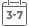# Introduction to Nonparametric Regression

• Publish Date: 2005-11-25
• Binding: Hardcover
• Author: K. Takezawa
• Sale
• \$195.24
• Regular price \$236.81An easy-to-grasp introduction to nonparametric regression

This book's straightforward, step-by-step approach provides an excellent introduction to the field for novices of nonparametric regression. Introduction to Nonparametric Regression clearly explains the basic concepts underlying nonparametric regression and features:
* Thorough explanations of various techniques, which avoid complex mathematics and excessive abstract theory to help readers intuitively grasp the value of nonparametric regression methods
* Statistical techniques accompanied by clear numerical examples that further assist readers in developing and implementing their own solutions
* Mathematical equations that are accompanied by a clear explanation of how the equation was derived

The first chapter leads with a compelling argument for studying nonparametric regression and sets the stage for more advanced discussions. In addition to covering standard topics, such as kernel and spline methods, the book provides in-depth coverage of the smoothing of histograms, a topic generally not covered in comparable texts.

With a learning-by-doing approach, each topical chapter includes thorough S-Plus? examples that allow readers to duplicate the same results described in the chapter. A separate appendix is devoted to the conversion of S-Plus objects to R objects. In addition, each chapter ends with a set of problems that test readers' grasp of key concepts and techniques and also prepares them for more advanced topics.

This book is recommended as a textbook for undergraduate and graduate courses in nonparametric regression. Only a basic knowledge of linear algebra and statistics is required. In addition, this is an excellent resource for researchers and engineers in such fields as pattern recognition, speech understanding, and data mining. Practitioners who rely on nonparametric regression for analyzing data in the physical, biological, and social sciences, as well as in finance and economics, will find this an unparalleled resource.

MORE FROM THIS COLLECTION